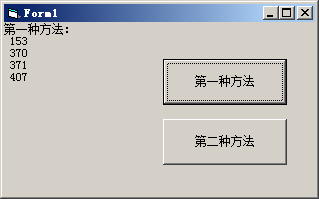﻿ VB程序题：编一程序，显示出所有的水仙花数。所谓水仙花数， (两种方法 龚沛曾课后答案) - 何老师的济亨网

# VB程序题：编一程序，显示出所有的水仙花数。所谓水仙花数， (两种方法 龚沛曾课后答案)

###### 2017-05-16 11:20:58  分类: VB程序题  参与: AddViewCount(75)人

VB程序题：编一程序，显示出所有的水仙花数。所谓水仙花数，是指一个3位数，其各位数字立方和等于该数字本身。例如，153是水仙花数，因为 153 = 13+53+33

1.利用三重循环，将三个数连接成一个3位数再进行判断。
2.利用一重循环，将所有的三位数逐位分离后再进行判断。

Private Sub Command1_Click()
Cls
Print "第一种方法："
Dim a As Integer, b As Integer, c As Integer, d As Integer
For a = 1 To 9
For b = 0 To 9
For c = 0 To 9
d = 100 * a + b * 10 + c
If d = a ^ 3 + b ^ 3 + c ^ 3 Then Print d
Next c
Next b
Next a
End Sub

Private Sub Command2_Click()
Cls
Print "第二种方法："
For i = 100 To 999
ge = i Mod 10
shi = i \ 10 Mod 10
bai = i \ 100
If ge ^ 3 + shi ^ 3 + bai ^ 3 = i Then Print i
Next i
End SubVisual Basic程序设计教程(第3版) (龚沛曾等编)课后实验源码

<< 上一篇下一篇 >>